JEE  >  Solved Examples: Some Basic Concepts of Chemistry

# Solved Examples: Some Basic Concepts of Chemistry Notes | Study Additional Documents and Tests for JEE - JEE

## Document Description: Solved Examples: Some Basic Concepts of Chemistry for JEE 2022 is part of Documents for Additional Documents and Tests for JEE preparation. The notes and questions for Solved Examples: Some Basic Concepts of Chemistry have been prepared according to the JEE exam syllabus. Information about Solved Examples: Some Basic Concepts of Chemistry covers topics like and Solved Examples: Some Basic Concepts of Chemistry Example, for JEE 2022 Exam. Find important definitions, questions, notes, meanings, examples, exercises and tests below for Solved Examples: Some Basic Concepts of Chemistry.

Introduction of Solved Examples: Some Basic Concepts of Chemistry in English is available as part of our Additional Documents and Tests for JEE for JEE & Solved Examples: Some Basic Concepts of Chemistry in Hindi for Additional Documents and Tests for JEE course. Download more important topics related with Documents, notes, lectures and mock test series for JEE Exam by signing up for free. JEE: Solved Examples: Some Basic Concepts of Chemistry Notes | Study Additional Documents and Tests for JEE - JEE
 1 Crore+ students have signed up on EduRev. Have you?

Solved Objective

Ex.1 8 litre of H2 and 6 litre of Cl2 are allowed to react to maximum possible extent. Find out the final volume of reaction mixture. Suppose P and T remains constant throughout the course of reaction -

(A) 7 litre (B) 14 litre (C) 2 litre (D) None of these.

Sol. (B)

H2 Cl2 → 2 HCl

Volume before reaction 8 lit 6 lit 0

Volume after reaction 2 0 12

Volume after reaction

= Volume of H2 left Volume of HCl formed = 2 12 = 14 lit

Ex.2 Naturally occurring chlorine is 75.53% Cl35 which has an atomic mass of 34.969 amu and 24.47% Cl37 which has a mass of 36.966 amu. Calculate the average atomic mass of chlorine-

(A) 35.5 amu (B) 36.5 amu (C) 71 amu (D) 72 amu

Sol. (A)

Average atomic mass

=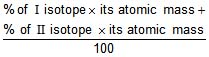=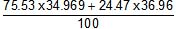= 35.5 amu.

Ex.3 Calculate the mass in gm of 2g atom of Mg-

(A) 12 gm (B) 24 gm (C) 6 gm (D) None of these.

Sol. (D)

Q 1 gm atom of Mg has mass = 24 gm

2 gm atom of Mg has mass = 24 x 2 = 48 gm.

Ex.4 In 5 g atom of Ag (At. wt. of Ag = 108), calculate the weight of one atom of Ag -

(A) 17.93 × 10-23gm (B) 16.93 × 10-23 gm

(C) 17.93 × 1023 gm (D) 36 × 10-23 gm

Sol. (A)

Q N atoms of Ag weigh 108 gm

1 atom of Ag weigh =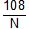=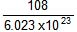= 17.93 × 10-23 gm.

Ex.5 In 5g atom of Ag (at. wt. = 108), calculate the no. of atoms of Ag -

(A) 1 N (B) 3N (C) 5 N (D) 7 N.

Sol. (C)

Q 1 gm atom of Ag has atoms = N

5 gm atom of Ag has atoms = 5N.

Ex.6 Calculate the mass in gm of 2N molecules of CO2 -

(A) 22 gm (B) 44 gm (C) 88 gm (D) None of these.

Sol. (C)

Q N molecules of CO2 has molecular mass = 44.

2N molecules of CO2 has molecular mass = 44 x 2 = 88 gm.

Ex.7 How many carbon atoms are present in 0.35 mol of C6H12O6 -

(A) 6.023 × 1023 carbon atoms (B) 1.26 × 1023 carbon atoms

(C) 1.26 × 1024 carbon atoms (D) 6.023 × 1024 carbon atoms

Sol. (C)

1 mol of C6H12O6 has = 6 N atoms of C

0.35 mol of C6H12O6 has = 6 × 0.35 N atoms of C

= 2.1 N atoms = 2.1 × 6.023 × 1023  = 1.26 × 1024 carbon atoms

Ex.8 How many molecules are in 5.23 gm of glucose (C6H12O6) -

(A) 1.65 × 1022 (B) 1.75 × 1022 (C) 1.75 × 1021 (D) None of these

Sol. (B)

Q 180 gm glucose has = N molecules

5.23 gm glucose has = = 1.75 × 1022 molecules

Ex.9 What is the weight of 3.01 × 1023 molecules of ammonia -

(A) 17 gm (B) 8.5 gm (C) 34 gm (D) None of these

Sol. (B)

Q 6.023 × 1023 molecules of NH3 has weight = 17 gm

3.01 × 1023  molecules of NH3 has weight

= = 8.50 gm

Ex.10 How many significant figures are in each of the following numbers -

(a) 4.003 (b) 6.023 × 1023 (c) 5000

(A) 3, 4, 1 (B) 4, 3, 2 (C) 4, 4, 4 (D) 3, 4, 3

Sol. (C)

Ex.11
How many molecules are present in one ml of water vapours at STP -

(A) 1.69 × 1019 (B) 2.69 × 10-19 (C) 1.69 × 10-19 (D) 2.69 × 1019

Sol. (D)

Q 22.4 litre water vapour at STP has

= 6.023 × 1023 molecules

1 × 10-3 litre water vapours at STP has

= × 10-3 = 2.69 × 10 19

Ex.12 How many years it would take to spend Avogadro's number of rupees at the rate of 1 million rupees in one second -

(A) 19.098 × 1019 years (B) 19.098 years

(C) 19.098 × 109 years (D) None of these

Sol. (C)

Q 106 rupees are spent in 1sec.

6.023 × 1023 rupees are spent in = sec

=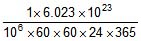years , = 19.098 × 109 year

Ex.13
An atom of an element weighs 6.644 × 10-23 g. Calculate g atoms of element in 40 kg-

(A) 10 gm atom (B) 100 gm atom (C) 1000 gm atom (D) 104 gm atom

Sol. (C)

Q weight of 1 atom of element

= 6.644 × 10-23 gm

weight of 'N' atoms of element

= 6.644 × 10-23 × 6.023 × 1023 = 40 gm

Q 40 gm of element has 1 gm atom.

40 x 103 gm of element has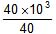, = 103 gm atom.

Ex.14 Calculate the number of Cl- and Ca 2 ions in 222 g anhydrous CaCl2 -

(A) 2N ions of Ca 2 4 N ions of Cl(B) 2N ions of Cl- & 4N ions of Ca 2

(C) 1N ions of Ca 2 & 1N ions of Cl- (D) None of these.

Sol. (A)

Q mol. wt. of CaCl2 = 111 g

Q 111 g CaCl2 has = N ions of Ca 2

222g of CaCl2 has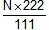= 2N ions of Ca 2

Also Q 111 g CaCl2 has = 2N ions of Cl-

222 g CaCl2 has =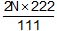ions of Cl-

= 4N ions of Cl- .

Ex.15 The density of O2 at NTP is 1.429g / litre. Calculate the standard molar volume of gas-

(A) 22.4 lit. (B) 11.2 lit (C) 33.6 lit (D) 5.6 lit.

Sol. (A)

Q 1.429 gm of O2 gas occupies volume = 1 litre.

32 gm of O2 gas occupies =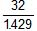,= 22.4 litre/mol.

Ex.16 Which of the following will weigh maximum amount-

(A) 40 g iron (B) 1.2 g atom of N

(C) 1 × 1023 atoms of carbon (D) 1.12 litre of O2 at STP

Sol. (A)

(A) Mass of iron=40g (B) Mass of 1.2 g atom of N = 14 × 1.2 = 16.8 gm

(D) Mass of 1 × 1023 atoms of C =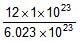= 1.99 gm.

(D) Mass of 1.12 litre of O2 at STP =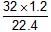= 1.6 g

Ex.17 How many moles of potassium chlorate to be heated to produce 11.2 litre oxygen -

(A) mol (B) mol (C) mol (D) mol.

Sol. (B)

2 KClO3 → 2KCl 3O2

Mole for reaction 2 2 3

Q 3 × 22.4 litre O2 is formed by 2 mol KClO3

11.2 litre O2 is formed by = mol KClO3

Ex.18 Calculate the weight of lime (CaO) obtained by heating 200 kg of 95% pure lime stone (CaCO3).

(A) 104.4 kg (B) 105.4 kg (C) 212.8 kg (D) 106.4 kg

Sol. (D)

Q 100 kg impure sample has pure

CaCO3 = 95 kg

200 kg impure sample has pure CaCO3

= = 190 kg. CaCO3 → CaO CO2

Q 100 kg CaCO3 gives CaO = 56 kg.

190 kg CaCO3 gives CaO = = 106.4 kg.

Ex.19 The chloride of a metal has the formula MCl3. The formula of its phosphate will be-

(A) M2PO4 (B) MPO4 (C) M3PO4 (D) M(PO4)2

Sol. (B) AlCl3 as it is AlPO4

Ex.20 A silver coin weighing 11.34 g was dissolved in nitric acid. When sodium chloride was added to the solution all the silver (present as AgNO3) was precipitated as silver chloride. The weight of the precipitated silver chloride was 14.35 g. Calculate the percentage of silver in the coin

(A) 4.8 % (B) 95.2% (C) 90 % (D) 80%

Sol. (B)

Ag 2HNO3 → AgNO3 NO2 H2O

108

AgNO3 NaCl → AgCl NaNO3

143.5

143.5 gm of silver chloride would be precipitated by 108 g of silver.

or 14.35 g of silver chloride would be precipitated 10.8 g of silver.

Q 11.34 g of silver coin contain 10.8 g of pure silver.

100 g of silver coin contain × 100 = 95.2 %.Solved Subjective

Ex.1 Calculate the following for 49 gm of H2SO4

(a) moles (b) Molecules (c) Total H atoms (d) Total O atoms

(e) Total electrons

Sol. Molecular wt of H2SO4 = 98

(a) moles =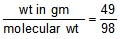=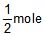(b) Since 1 mole = 6.023 × 1023 molecules.= 6.023 × 1023 × molecules = 3.011 × 1023 molecules

(c) 1 molecule of H2SO4 Contains 2 H atom

3.011 × 1023 of H2SO4 contain 2 × 3.011 × 1023 atoms = 6.023 × 1023 atoms

(d) 1 molecules of H2SO4 contains 4 O atoms

3.011 × 1023 molecular of H2SO4 contains = 4 × 3.011 × 1023 = 12.044 × 1023

(e) 1 molecule of H2SO4 contains 2H atoms 1 S atom 4 O atom

this means 1 molecule of H2SO4 Contains (2 16 4 × 8) e-

So 3.011 × 1023 molecules have 3.011 × 1023 × 50 electrons = 1.5055 × 1025 e-

Ex.2 Calculate the total ions & charge present in 4.2 gm of N-3

Sol. mole =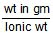=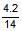= 0.3

total no of ions = 0.3 × NA ions

total charge = 0.3 NA × 3 × 1.6 × 10-19

= 0.3 × 6.023 × 1023 × 3 × 1.6 × 10-19 , = 8.67 × 104 C Ans.

Ex.3 Find the total number of iron atom present in 224 amu iron.

Sol. Since 56 amu = 1 atom
therefore 224 amu =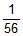× 224 = 4 atom Ans.

Ex.4 A compound containing Ca, C, N and S was subjected to quantitative analysis and formula mass determination. A 0.25 g of this compound was mixed with Na2CO3 to convert all Ca into 0.16 g CaCO3. A 0.115 gm sample of compound was carried through a series of reactions until all its S was changed into SO42- and precipitated as 0.344 g of BaSO4. A 0.712 g sample was processed to liberated all of its N as NH3 and 0.155 g NH3 was obtained. The formula mass was found to be 156. Determine the empirical and molecular formula of the compound.

Sol. Moles of CaCO3 =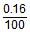= Moles of Ca

Wt of Ca =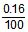× 40

Mass % of Ca =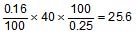Similarly Mass % of S =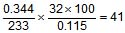Similarly Mass % of N = = 17.9

⇒ Mass % of C = 15.48

Now :

Elements Ca S N C

Mass % 25.6 41 17.9 15.48

Mol ratio 0.64 1.28 1.28 1.29

Simple ratio 1 2 2 2

Empirical formula = CaC2N2S2,

Molecular formula wt = 156 , n × 156 = 156 ⇒ n = 1

Hence, molecular formula = CaC2N2S2

Ex.5 A polystyrne having formula Br3C6H3(C3 H8)n found to contain 10.46% of bromine by weight. Find the value of n. (At. wt. Br = 80)

Sol. Let the wt of compound is 100 gm & molecular wt is M

Then moles of compound =

Moles of Br = × 3

wt of Br = × 3 × 80 = 10.46

M = 2294.45 = 240 75 44 n , Hence n = 45 Ans.

Ex.6 A sample of clay was partially dried and then analysed to 50% silica and 7% water. The original clay contained 12% water. Find the percentage of silica in the original sample.

Sol. In the partially dried clay the total percentage of silica water = 57%. The rest of 43% must be some impurity. Therefore the ratio of wts. of silica to impurity = . This would be true in the original sample of silica.

The total percentage of silica impurity in the original sample is 88. If x is the percentage of silica, ; x = 47.3% Ans.

Ex.7 A mixture of CuSO4.5H2O and MgSO4. 7H2O was heated until all the water was driven-off. if 5.0 g of mixture gave 3 g of anhydrous salts, what was the percentage by mass of CuSO4.5H2O in the original mixture ?

Sol. Let the mixture contain x g CuSO4.5H2O

⇒ = 3 ⇒ x = 3.56

⇒ Mass percentage of CuSO4. 5H2O = = 71.25 % Ans.

Ex .8
367.5 gm KClO3 (M = 122.5) when heated, How many litre of oxygen gas is proudced at S.T.P.

Sol. KClO3 → KCl O2

Applying POAC on O, moles of O in KClO3 = moles of O in O2

3 × moles of KClO3 = 2 × moles of O2

3 × = 2 × n, n = ×

Volume of O2 gas at S.T.P = moles × 22.4

=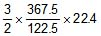= 9 × 11.2 = 100.8 lit Ans.

Ex.9 0.532 g of the chloroplatinate of a diacid base on ignition left 0.195 g of residue of Pt. Calculate molecular weight of the base (Pt = 195)

Sol. Suppose the diacid base is B.

B H2PtCl6→ BH2PtCl6 → Pt

diacid acid chloroplatinate

base 0.532 g 0.195 g

Since Pt atoms are conserved, applying POAC for Pt atoms,

moles of Pt atoms in BH2PtCl6 = moles of Pt atoms in the product

1 × moles of BH2PtCl6 = moles of Pt in the product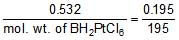mol. wt. of BH2PtCl6 = 532

From the formula BH2PtCl6, we get

mol. wt. of B = mol. wt. of BH2PtCl6 - mol. wt. of H2PtCl6

= 532 - 410 = 122. Ans.

Ex.10 10 mL of a gaseous organic compound containing. C, H and O only was mixed with 100 mL of oxygen and exploded under conditions which allowed the water formed to condense. The volume of the gas after explosion was 90 mL. On treatment with potash solution, a further contraction of 20 mL in volume was observed. Given that the vapour density of the compound is 23, deduce the molecular formula. All volume measurements were carried out under the same conditions.

Sol. CxHyOz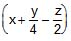O2 → xCO2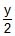H2O

10 ml after explosion volume of gas = 90 ml

90 = volume of CO2 gas volume of unreacted O2

on treatment with KOH solution volume reduces by 20 ml. This means the volume of CO2 = 20 ml

the volume of unreacted O2 = 70 ml

volume of reacted O2 = 30 ml

V.D of compoud = 23

molecular wt 12x y 16z = 46 ...(1)

from equation we can write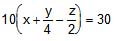, x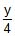-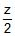= 3

4x y - 2z = 12 ...(2)

& 10x = 20 ⇒ x = 2

from eq. (1) & (2) ; z = 1 & y = 6; Hence C2H6O Ans.

The document Solved Examples: Some Basic Concepts of Chemistry Notes | Study Additional Documents and Tests for JEE - JEE is a part of the JEE Course Additional Documents and Tests for JEE.
All you need of JEE at this link: JEE

## Additional Documents and Tests for JEE

11 videos|159 docs|14 tests
 Use Code STAYHOME200 and get INR 200 additional OFF

## Additional Documents and Tests for JEE

11 videos|159 docs|14 tests

Track your progress, build streaks, highlight & save important lessons and more!

,

,

,

,

,

,

,

,

,

,

,

,

,

,

,

,

,

,

,

,

,

;